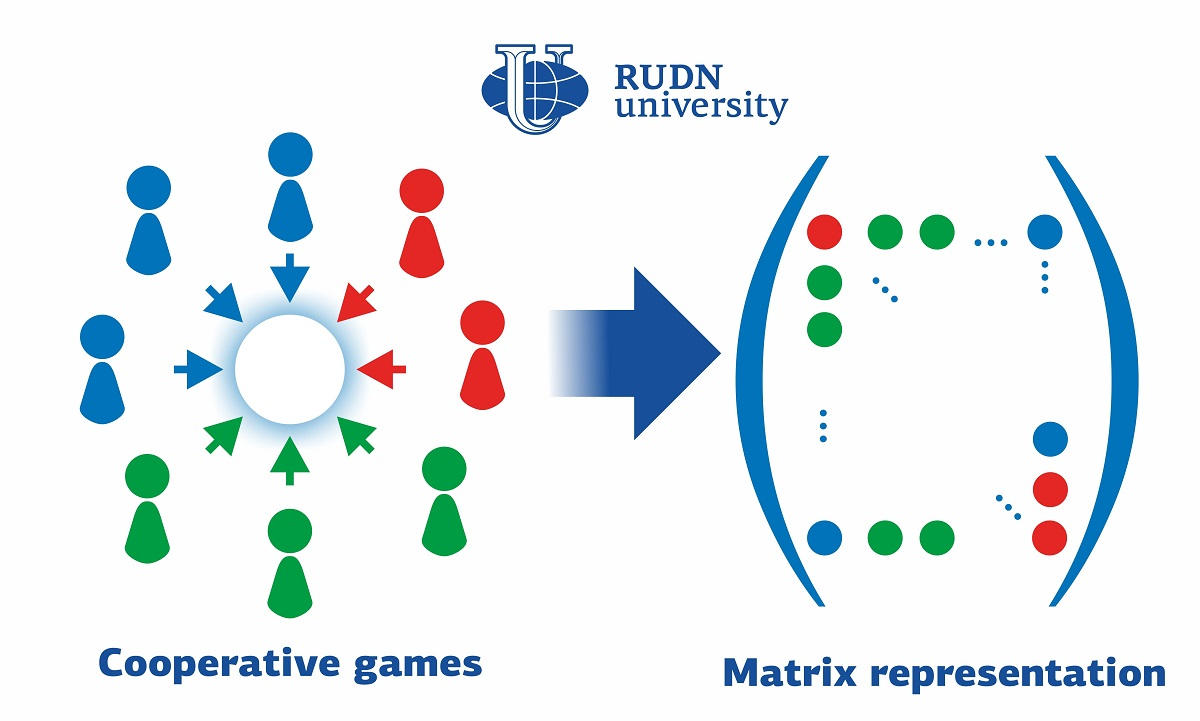RUDN University Mathematician Suggested New Approach to Cooperative Game

# RUDN University Mathematician Suggested New Approach to Cooperative Game

A mathematician from RUDN University developed a matrix representation of set functions. This approach is vivid and easy to check, and it makes the calculations easier. Among other things, the new development can be applied to cooperative game theory.

Specialists in cooperative game theory study methods of complex decision-making in situations with multiple criteria. In such a situation, groups (or coalitions) of players have to come up with a decision that is the most profitable for all of them. Set functions are one of the tools used to work with cooperative game theory. In these functions, the input data are sets of elements that can have different values. Simple explicit questions are quite rare in real life; therefore, the data on different elements can support or neutralize each other. Combinations of elements called coalitions can assume their own values. To work with this apparatus, scientists require an intuitive mathematical language. A mathematician from RUDN University suggested his approach to it

“Our contribution to the mathematical language of cooperative game theory is based on the familiar notions of matrices and vectors. We have developed a formal approach to manipulations with set functions based on linear algebra. Our results can be practically applied to multicriteria decision analysis, group decision-making, operations with dependent goals, economic theories based on cooperative games, and aggregate functions theory,” said Prof. Gleb Beliakov, a Candidate of Physics and Mathematics from RUDN University.

Prof. Beliakov wanted to develop a universal approach that would make expressions equally understandable and convenient for mathematicians, engineers, economists, and specialists in computer science. The best option for it was linear algebra operations based on matrices. Operations with matrices are included in most software packages and are also useful for parallel computations

The scientist obtained matrix expressions by transforming a derived set function expression. A derived function shows how a function transforms when its variables change. Having calculated a derived function, a specialist can give an accurate analysis of a certain situation. In linear algebra, treating an exponential set this way can simplify calculation methods and support effective implementation of many formulae in software. Prof. Beliakov also suggested new formulae for finding the Shapley vector—a version of ‘fair division’ in which the profit of each player is equal to their average contribution to respective coalitions. The new method makes it easier to obtain the Shapley vector in practical applications.

“Set functions are used in economics, decision-making, fuzzy logic, and operational research. An exponential set is a particularly effective tool to model input variables in corporate games. The new apparatus could simplify calculations and support software implementation of many formulae using existing linear algebra packages,” added Prof. Gleb Beliakov from RUDN University.

The results of the work were published in the Information Sciences journal.News
All news
Science
20 Sep
RUDN University mathematician determined the conditions for the coexistence of three species in the wild

RUDN University mathematician together with colleagues from India and France for the first time studied in detail the system of coexistence of three species of living creatures in the wild. The results help to understand what parameters determine the extinction andли survivalof species, and how the number of species changes in space and time.

Science
15 Sep
RUDN University Chemist Created Coordination Polymers Films with up to 99.99% antibacterial efficiency

RUDN University chemist with his colleagues from Portugal has developed two types of coating based on new coordination polymers with silver. Both compounds were successfully tested against four common pathogens.

Science
12 Sep
RUDN scientists have improved titanium dental implants with graphene nanosloyers

RUDN researchers have created and tested a method for processing titanium dental implants. It turned out that theanoslos of graphene on the surface of titanium improve its interaction with stem cells,which are placed on the implant so that it better "takes root".Thanks to this method ofprocessing, stem cells are better kept on the surface, multiply and turn into the desired cells.